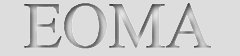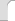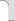Eastern Oregon Mining AssociationServing the mineral industriesPlacer · Lode · Prospecting · Filing ·Weights & Formulas· GlossaryWEIGHT CONVERSIONS FOR GOLD, SILVER AND GEMS:Gold and silver are measured in Troy weight, a system that includes pennyweights, ounces and pounds. The ounces and pounds do not equal the Avordupois or customary U.S. system that other common goods are measured in. For example a troy ounce weighs about 10 percent more than a Avordupois ounce. * 24 grains = 1 pennyweight = 1.5552 grams * 1 gram = .64 pennyweight * 1 pennyweight = 1.56 grams or .05 troy ounce * 20 pennyweight = 1 troy ounce = 31.1035 grams * 12 ounces = 1 pound troy = 373.24 grams. TO CONVERT:* Avordupois Ounces to Troy ounces multiply by .91 * Avordupois Ounces to pennyweight multiply by 18.2 * Avordupois ounces to grams multiply by 28.35 * Troy ounces to grams multiply by 31.1 * Troy ounces to pennyweight multiply by 20 * Troy ounces to pounds troy, divide by 12 * Pennyweight to grams multiply by 1.56 * Pennyweight to troy ounces multiply by .05 (or divide by 20) * Grams to pennyweight multiply by .64 * Grams to troy ounces multiply by .032 * Grams to carats multiply by 5 * Carats to grams multiply by .2 (or divide by 5) * Carats to "Points" multiply by 100. * "Points" to carats multiply by .01 (or divide by 100) SPECIFIC GRAVITY FORMULA Specific gravity is a measure of the density of a mineral. At times it is such a useful property that it is the only way to distinguish some minerals without laboratory or optical techniques. Gold can easily be distinguished from "fool's gold" by specific gravity alone, although there are many other ways. Specific gravity is a unitless measure, because it is derived from the density of the mineral divided by the density of water and thus all units cancel. However, since water's density equals 1 gram per cubic centimeter (at specific conditions), then a mineral's specific gravity would also correspond to a mineral's density as expressed in grams per cubic centimeter. Specific gravity, or SG, as already stated compares the density of a mineral to the density of water. If a mineral has a SG of 2, then it is twice as dense as water. If a mineral has a SG of 3 then it is three times as dense as water and so forth. However, comparing a mineral to water is not practical and is not really helpful. It is easier to consider what is the SG of a typical mineral and compare minerals that way. The Specific Gravity Test (used to calculate the gold content of gold nuggets that contain a mixture of gold and other materials) In this example of the specific gravity test, we use gold mixed with quartz. Simply put, the formula is 3.1 x the weight in water, minus 1.9 x the weight in air: Wet nugget weighs 74.5 grams x 3.1 = 230.95 Dry nugget weighs 96 grams x 1.9 = 182.4 230.95 - 182.4 = 48.55 31.1 = number of grams per ounce 48.55 / 31.1 = 1.56 ounces of gold Original dry weight = 96 grams / 31.1 = 3.08 ounces. Subtract the difference between the wet and the dry: 3.08 ounces - 1.56 ounces = 1.52 ounces. Therefore what is left is 1.52 ounces of quartz. "Wet" = weight in water - Put container of water (enough water to cover nugget) on scale. Tare (zero) out scale. Hang nugget by string in water. Note weight. The specific gravity for gold is 19.3. The specific gravity for quartz is 2.65. The ratio between gold and quartz is 7.28. Tip from Miner X: "For the wet weight, what I do with digital scales is to put two boards on the rim of a bucket filled with water, with a gap between them. Set the scales on the boards, tie a string around the ore sample and loop it over the pan so that the sample is suspended below, in the bucket, hanging freely. Record the weight, then weigh the string separately and subtract it from the total. All you have to do then is weigh the sample dry, on its own, and use the formula above."